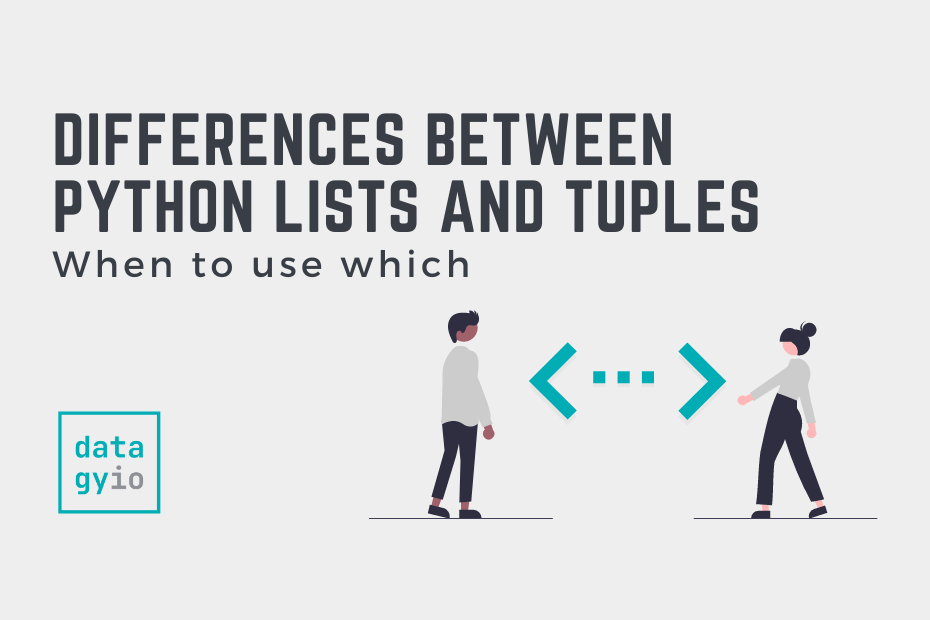# Python: Differences Between Lists and TuplesIn this tutorial, you’ll learn the differences between Python lists and tuples. Lists and tuples are fundamental Python container data structures. On the surface, they seem very similar. However, there are a number of unique differences between them that makes their use cases quite clear.

By the end of this tutorial, you’ll have learned:

• What the key differences and similarities are between Python Lists and Python Tuples
• When using a list is more appropriate than using a tuple
• When using a tuple is more appropriate than using a list
• What methods are and aren’t available for the two data structures

While both lists and tuples are container data structures in Python, they have unique attributes. Python lists, for example, can be helpful to store values that need to be modified, deleted, or added to. Inversely, Python tuples allow you to rest easy knowing that data in them cannot be modified.

## Key Differences Between Python Lists and Python Tuples

The table below breaks down the key differences and similarities between Python lists and tuples. Some of the items will be true for both lists and tuples, while others will have their differences noted:

In the following sections, we’ll break these points down in more detail. There are some technical complexities to each of these items and many deserve a bit of a deeper dive.

## Creating and Indexing Python Lists and Tuples

Python lists and tuples works very similarly. Python lists use square brackets `[]`, while Python tuples use regular parentheses `()`. Both of these can contain different data types, meaning that they are heterogeneous.

Let’s take a look at how these two data types can be created:

``````# Creating Python Lists and Tuples
a_list = ['datagy', 1, True]
a_tuple = ('datagy', 1, True)``````

Because both of these objects are ordered, we can access items within them using their index position. Both positive and negative indices work.

Let’s try accessing a little bit of data in them:

``````# Accessing items in Python Lists and Tuples by their Index Position
print(a_list)
print(a_tuple[-1])

# Returns:
# datagy
# True``````

So far, these two objects seem quite similar. In the next section, we’ll dive into the mutability of them to better understand where some key differences lie.

## Mutability of Python Lists and Tuples

Python lists are mutable objects, meaning that you can update items in them, delete items in them, or add items to them.

Meanwhile, Python tuples are immutable: meaning that once they are created, they cannot be changed.

Let’s see how we can change the value in a Python list:

``````# Modifying a Python List's Values
a_list = ['datagy', 1, True]
a_list = False
print(a_list)

# Returns: ['datagy', 1, False]``````

Let’s see what happens when we try to do the same with our tuples:

``````# Attempting to modify a tuple's value
a_tuple = ('datagy', 1, True)
a_tuple = False
print(a_tuple)

# Raises: TypeError: 'tuple' object does not support item assignment``````

By attempting to modify a tuple’s values an error was raised.

There is one important exception to this. If our tuple contains a mutable object, such as a list, then that item can be modified. Let’s see what this looks like:

``````# Modifying a mutable item in a tuple
another_tuple = (1,2,[3,4])
another_tuple.append(5)

print(another_tuple)

# Returns: (1, 2, [3, 4, 5])``````

## Memory Consumption of Python Lists and Tuples

Because Python lists are mutable and Python tuples are not, Python is able to be more memory-efficient when working with tuples.

The reason for this is that when Python creates a list, it allocates extra space in order to be able to mutate it. On the other hand, with Python tuples, Python knows exactly how much memory to allocate!

In order to better illustrate this, let’s check the sizes of our tuple and list:

``````# Checking the sizes of tuples and lists
a_list = ['datagy', 1, True]
a_tuple = ('datagy', 1, True)

print(a_list.__sizeof__())
print(a_tuple.__sizeof__())

# Returns:
# 64
# 48``````

We can see that the list is 33% larger in bytes! At this scale, it may not make much of a difference. However, when you start working with larger datasets, the difference can be huge.

## Python List and Tuple Methods Compared

Because Python lists are mutable, they have many more methods available to them. That said, Python tuples also have a large number of methods that can help make finding and retrieving data easier.

Just by comparing the length of the tables will illustrate how many more methods are available for lists than tuples.

Let’s start by looking at Python list methods:

### Python List Methods

The following list contains all the methods available to Python lists:

Now let’s take a look at the methods available to tuples:

### Python Tuple Methods

The following methods are available for tuples:

## Copying Python Lists and Tuples

One interesting thing to note about tuples and lists in Python is that lists can be copied, while tuples cannot. Because tuples are immutable, when you try to copy a tuple it simply returns itself.

Let’s see what this looks like first with lists:

``````# Making a copy of a list
a_list = ['datagy', 1, True]
another_list = list(a_list)

print(id(a_list))
print(id(another_list))

# Returns:
# 140341145035072
# 140340875289536``````

We can see that these two lists point at different places in memory. Now, let’s try this with tuples:

``````# Copying tuples
a_tuple = ('datagy', 1, True)
another_tuple = tuple(a_tuple)

print(id(a_tuple))
print(id(another_tuple))

# Returns:
# 140324366994240
# 140324366994240``````

## Conclusion

In this tutorial, you learned about the differences between Python lists and tuples. Understanding the differences between these two data structures allows you to better understand when to use one over the other.

Some of the key differences are that tuples are immutable, have fixed lengths, and can be more memory efficient. Inversely, lists are mutable, don’t have fixed lengths and can use more memory.